# NCERT Solutions: Solutions - 1 Notes | Study Chemistry Class 12 - NEET

## NEET: NCERT Solutions: Solutions - 1 Notes | Study Chemistry Class 12 - NEET

The document NCERT Solutions: Solutions - 1 Notes | Study Chemistry Class 12 - NEET is a part of the NEET Course Chemistry Class 12.
All you need of NEET at this link: NEET

Q.1. Determine the osmotic pressure of a solution prepared by dissolving 25 mg of K2SO4 in 2 liter of water at 25° C, assuming that it is completely dissociated.
Ans. When K2SO4 is dissolved in water, K+ and SO42- ions are produced.
K2SO→ 2K+ + SO42-
Total number of ions produced = 3
i =3
Given,
w = 25 mg = 0.025 g
V = 2 L
T = 250C = (25 + 273) K = 298 K
Also, we know that:
R = 0.0821 L atm K-1mol-1
M = (2 × 39) (1 × 32) (4 × 16) = 174 g mol-1
Appling the following relation,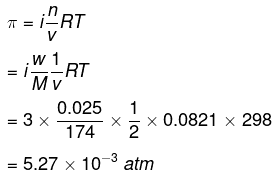Q.2. Calculate the mole fraction of benzene in solution containing 30% by mass in carbon tetrachloride.
Ans.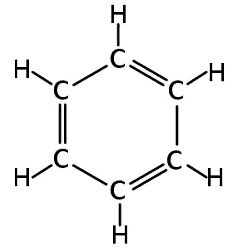Benzene

Let the total mass of the solution be 100 g and the mass of benzene be 30 g.
∴ Mass of carbon tetrachloride = (100 − 30)g = 70 g
Molar mass of benzene (C6H6) = (6 × 12 + 6 × 1) g mol−1 = 78 g mol−1
∴ Number of moles of C6H6 = 30 /78 mol = 0.3846 mol
Molar mass of carbon tetrachloride (CCl4) = 1 × 12 + 4 × 355 = 154 g mol−1
∴ Number of moles of CCl4 = 70/154 mol = 0.4545 mol
Thus, the mole fraction of C6H6 is given as: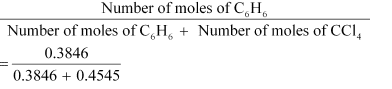= 0.458

Q.3. Calculate the molarity of each of the following solutions:
(a) 30 g of Co(NO3)2. 6H2O in 4.3 L of solution
(b) 30 mL of 0.5 M H2SO4 diluted to 500 mL.
Ans. Molarity is given by:
Molarity = Moles of solute/Volume of solution in litre
(a) Molar mass of Co (NO3)2.6H2O = 59 + 2 (14 + 3 × 16) + 6 × 18 = 291 g mol−1
∴ Moles of Co (NO3)2.6H2O = 30 / 291 mol = 0.103 mol
Therefore, molarity = 0.103 mol/4.3 L = 0.023 M
(b) Number of moles present in 1000 mL of 0.5 M H2SO4 = 0.5 mol
∴ Number of moles present in 30 mL of 0.5 M H2SO4 = 0.5 × 30/1000 mol = 0.015 mol
Therefore, molarity = 0.015/0.5 L mol = 0.03 M

Q.4. 19.5 g of CH2FCOOH is dissolved in 500 g of water. The depression in the freezing point of water observed is 1.0°C. Calculate the van’t Hoff factor and dissociation constant of fluoroacetic acid.
Ans. It is given that: w= 500g
w2 = 19.5g
K= 1.86 K kg mol-1
ΔTf = 1 K
We know that: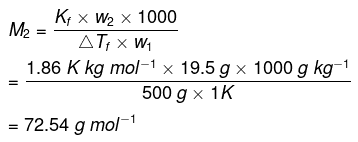Therefore, observed molar mass of
CH2FCOOH, (M2)obs = 72.54 g mol
The calculated molar mass of CH2FCOOH is:
(M2)cal = 14 + 19 + 12 + 16 + 16 + 1
=78 g mol-1
Therefore,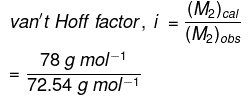=1.0753
Let α be the degree of dissociation of CH2FCOOH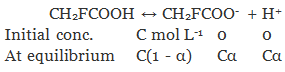Total = C(1 + α)
⇒ i = 1 + α
⇒ α = i - 1
= 1.0753 - 1
= 0.0753
Now, the value of Ka is given as: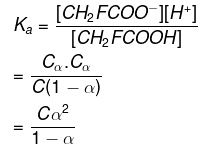Taking the volume of the solution as 500 mL, we have the concentration: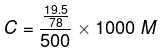= 0.5 M
Therefore,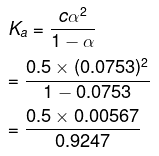= 0.00307 (approximately)
= 3.07 × 10-3

Q.5. Calculate (a) molality (b) molarity and (c) mole fraction of KI if the density of 20% (mass/mass) aqueous KI is 1.202 g mL-1.
Ans.
(a) Molar mass of KI = 39 + 127 = 166 g mol−1
20% (mass/mass) aqueous solution of KI means 20 g of KI is present in 100 g of solution.
That is,
20 g of KI is present in (100 − 20) g of water = 80 g of water
Therefore,
Molality of the solution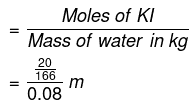= 1.506 m
= 1.51 m (approximately)
(b) It is given that the density of the solution = 1.202 g mL−1
∴ Volume of 100 g solution = Mass / Density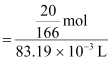= 83.19 mL
= 83.19 × 10−3 L
Therefore, molarity of the solution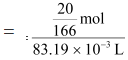= 1.45 M

(c) Moles of Kl = 20/166 = 0.12 mol
Moles of water = 80/18 = 4.44
Therefore, mole fraction of Kl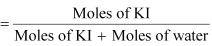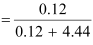= 0.0263

Q.6. H2S, a toxic gas with rotten egg like smell, is used for the qualitative analysis. If the solubility of H2S in water at STP is 0.195 m, calculate Henry’s law constant.
Ans.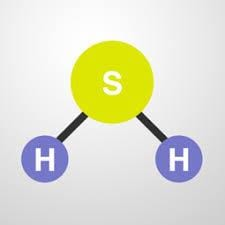H2SIt is given that the solubility of H2S in water at STP is 0.195 m, i.e., 0.195 mol of H2S is dissolved in 1000 g of water.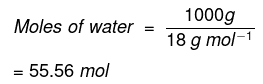∴ Mole fraction of H2S,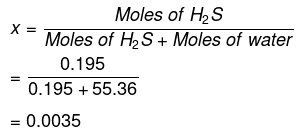At STP, pressure (p) = 0.987 bar
According to Henry’s law:
p = KHx
= 0.987 / 0.0035 Bar
= 282 bar

Q.7. Henry’s law constant for CO2 in water is 1.67 × 108 Pa at 298 K. Calculate the quantity of CO2 in 500 mL of soda water when packed under 2.5 atm CO2 pressure at 298 K.
Ans. It is given that: KH = 1.67 × 108 Pa
pco2 = 2.5 atm = 2.5 × 1.01325 × 105 Pa
= 2.533125 × 105 Pa
According to Henry’s law: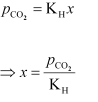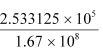= 0.00152
We can write,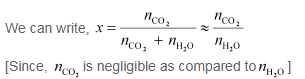[Since,  is negligible as compared to ]
In 500 mL of soda water, the volume of water = 500 mL
[Neglecting the amount of soda present]
We can write:
500 mL of water = 500 g of water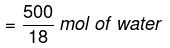= 27.78 mol of water
Now,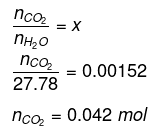Hence, quantity of CO2 in 500 mL of soda water = (0.042 × 44)g
= 1.848 g

Q.8. The vapour pressure of pure liquids A and B are 450 and 700 mm Hg respectively, at 350 K. Find out the composition of the liquid mixture if total vapour pressure is 600 mm Hg. Also find the composition of the vapour phase.
Ans. It is given that: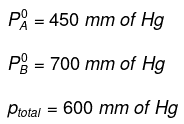from Rault's law, we have: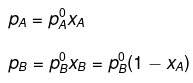Therefore, total pressure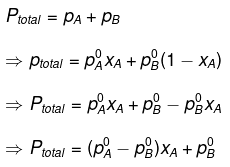⇒ 600 = (450 - 700) xA + 700
⇒ - 100 = -250xA
⇒ xA = 0.4
Therefore, xB  = 1 - xA
= 1 - 0.4
= 0.6
Now,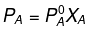= 450 × 0.4
= 180 mm of Hg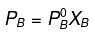= 700 × 0.6
= 420 mm of Hg
Now, in the vapour phase:
Mole fraction of liquid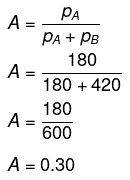And, mole fraction of liquid B = 1 − 0.30 = 0.70

Q.9. Vapour pressure of pure water at 298 K is 23.8 mm Hg. 50 g of urea (NH2CONH2) is dissolved in 850 g of water. Calculate the vapour pressure of water for this solution and its relative lowering.
Ans. It is given that vapour pressure of water,  p01 = 23.8 mm of Hg
Weight of water taken, w1 = 850 g
Weight of urea taken, w2 = 50 g
Molecular weight of water, M1 = 18 g mol−1
Molecular weight of urea, M2 = 60 g mol−1
Now, we have to calculate vapour pressure of water in the solution. We take vapour
pressure as p1.
Now, from Raoult’s law, we have: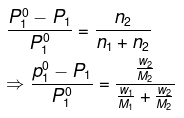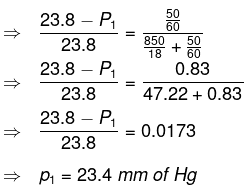Hence, the vapour pressure of water in the given solution is 23.4 mm of Hg and its relative lowering is 0.0173.

Q.10. Boiling point of water at 750 mm Hg is 99.63°C. How much sucrose is to be added to 500 g of water such that it boils at 100°C. Molal elevation constant for water is 0.52 K kg mol−1.
Ans. Here, elevation of boiling point
ΔTb = (100 273) − (99.63 273)
= 0.37 K
Mass of water, wl = 500 g
Molar mass of sucrose (C12H22O11), M2 = 11 × 12 + 22 × 1 + 11 × 16
= 342 g mol−1
Molal elevation constant, Kb = 0.52 K kg mol−1
We know that: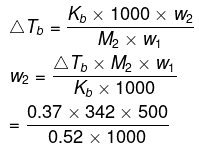= 121.67 g (approximately)
Hence, 121.67 g of sucrose is to be added.
Note: There is a slight variation in this answer and the one given in the NCERT textbook.

Q.11. Determine the amount of CaCl2 (i = 2.47) dissolved in 2.5 litre of water such that its osmotic pressure is 0.75 atm at 27°C.
Ans.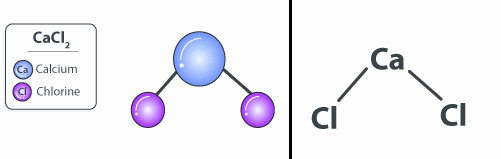Structure of CaCl2We know that,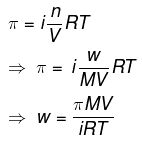π = 0.75 atm
V = 2.5 L
i = 2.47
T = 300 K
Here,
R = 0.0821 L atm K-1mol-1
M = 1 × 40.2 × 35.5
= 111 g mol-1
Therefore,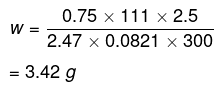Hence, the required amount of CaCl2 is 3.42 g.

Q.12. Vapour pressure of water at 293 K is 17.535 mm Hg. Calculate the vapour pressure of water at 293 K when 25 g of glucose is dissolved in 450 g of water.
Ans. Vapour pressure of water, p°1 = 17.535 mm of Hg
Mass of glucose, w2 = 25 g
Mass of water, w1 = 450 g
We know that,
Molar mass of glucose (C6H12O6), M2 = 6 × 12 + 12 × 1 + 6 × 16
= 180 g mol−1
Molar mass of water, M1 = 18 g mol−1
Then, number of moles of glucose,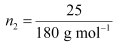= 0.139 mol
And, number of moles of water,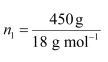= 25 mol
We know that,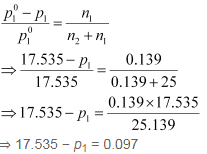⇒ p1 = 17.44 mm of Hg
Hence, the vapour pressure of water is 17.44 mm of Hg.

Q.13. Define the term solution. How many types of solutions are formed? Write briefly about each type with an example.
Ans. Homogeneous mixtures of two or more than two components are known as solutions.
There are three types of solutions.
(i) Gaseous solution: The solution in which the solvent is a gas is called a gaseous solution. In these solutions, the solute may be liquid, solid, or gas.
Example: a mixture of oxygen and nitrogen gas is a gaseous solution.
(ii) Liquid solution: The solution in which the solvent is a liquid is known as a liquid solution. The solute in these solutions may be gas, liquid, or solid.
Example: a solution of ethanol in water is a liquid solution.
(iii) Solid solution: The solution in which the solvent is a solid is known as a solid solution. The solute may be gas, liquid or solid. For example, a solution of copper in gold is a solid solution.

Q.14. Give an example of solid solution in which the solute is a gas.
Ans. In case a solid solution is formed between two substances (one having very large particles and the other having very small particles), an interstitial solid solution will be formed.
Example: a solution of hydrogen in palladium is a solid solution in which the solute is a gas.

The document NCERT Solutions: Solutions - 1 Notes | Study Chemistry Class 12 - NEET is a part of the NEET Course Chemistry Class 12.
All you need of NEET at this link: NEETUse Code STAYHOME200 and get INR 200 additional OFF

## Chemistry Class 12

111 videos|306 docs|259 tests

### How to Prepare for NEET

Read our guide to prepare for NEET which is created by Toppers & the best Teachers

Track your progress, build streaks, highlight & save important lessons and more!

,

,

,

,

,

,

,

,

,

,

,

,

,

,

,

,

,

,

,

,

,

;